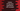# Python string join() method explanation with example## How to use python string join() method:

join() is a method defined in the string class in python. This method is used to concatenate all strings in an iterable. This method is really useful if you want to create a new string by concatenating all values of an iterable. For example, if you want to concatenate all characters of a list to create a new string, you can use join.

In this post, we will learn how to use join with different examples.

### Definition of join():

join is defined as below:

``str.join(iterable)``

Here,

• iterable is the iterable containing strings.

The separators between the elements is the string provided.

If the iterable contains any non-string value, it throws one TypeError.

### Example of join():

Let’s try with a list of strings first:

#### Example 1:

``````new_string = ' '.join(['Hello','World','!!'])

print(new_string)``````

It is joining all strings with a space in between the strings.

It will print the below output:

``Hello World !!``

#### Example 2:

Now, let’s try to join the strings with a different separator:

``````new_string = ','.join(['Hello','World','!!'])

print(new_string)``````

It is separating the strings by comma. It will print:

``Hello,World,!!``

#### Example 3:

We can use any string we want as the separator:

``````new_string = '####'.join(['Hello','World','!!'])

print(new_string)``````

It gives:

``Hello####World####!!``

#### Example 4:

join can be used to modify a string. For example, the below example changes the string by separating each character by ,.

``````new_string = ','.join('abcdef')

print(new_string)``````

It will print:

``a,b,c,d,e,f``